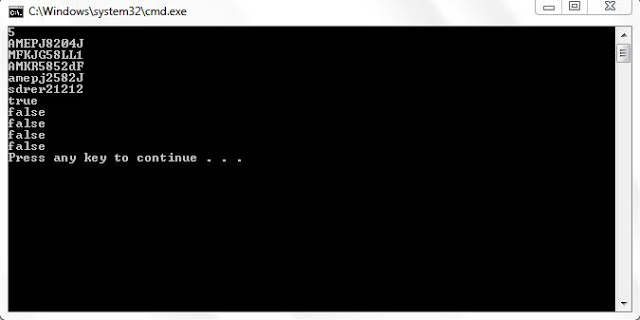## Java program to validate the PAN card Number : Regular expression

Write a java program to validate a PAN Card number. A PAN card number is said to be valid when it starts with 5 uppercase letters 4 digits and Followed by single upper case letter. A Valid PAN have only 10 letters.

For example: a pan card number AMEPJ2585B is valid where as AM525LDKF is invalid.

Given below is the java program which makes use of regular expressions to validate the PAN number.

ValidPan.java

```import java.util.*;
class ValidPAN
{
public static void main(String[] args)
{
Scanner scn=new Scanner(System.in);
int t=scn.nextInt();
String s[]=new String[t];
boolean output[]=new boolean[t];
for(int i=0; i<s.length;i++)
{
s[i]=scn.next();
boolean ss=s[i].matches("[A-Z]{5}\\d{4}[A-Z]{1}");
output[i]=ss;
}
for(boolean b:output)
{
System.out.println(b);
}

}
}
```
```
```

```
```
`Sample Output: `## Java program to convert the number in time format

Given below is the java program to print the time given in number format to String format.

For example: if you give input as 5 45, the below program will print Quarter to six

```import java.util.*;
class TimeTest
{
public static void main(String[] args)
{
HashMap<Integer,String> hm=new HashMap<Integer,String>();
hm.put(1,"one");hm.put(2,"two");hm.put(3,"three");hm.put(4,"four");hm.put(5,"five");
hm.put(6,"six");hm.put(7,"seven");hm.put(8,"eight");hm.put(9,"nine");hm.put(10,"ten");
hm.put(11,"eleven");hm.put(12,"twelve");hm.put(13,"thirteen");hm.put(14,"forteen");hm.put(15,"fifteen");
hm.put(16,"sixteen");hm.put(17,"seventeen");hm.put(18,"eighteen");hm.put(19,"ninteen");hm.put(20,"twenty");
hm.put(21,"twenty one");hm.put(22,"twenty two");hm.put(23,"twenty three");hm.put(24,"twenty four");hm.put(25,"twenty five");
hm.put(26,"twenty six");hm.put(27,"twenty seven");hm.put(28,"twenty eight");hm.put(29,"twenty nine");hm.put(30,"thirty");

Scanner scn=new Scanner(System.in);

int hour=scn.nextInt();
int minute=scn.nextInt();

if(minute==00)
{
}
else
if(minute==15)
{
}
else
if(minute==30)
{
}
else
if(minute>30&&minute==45)
{
}
else
if(minute>30&&minute!=45)
{
minute=60-minute;
String midString="minute";
String min=String.valueOf(minute);
if(min.length()>1)
{
midString="minutes";
}
addString=hm.get(minute)+" "+midString+" to "+hm.get(hour+1);
}

else
{
String midString="minute";
String min=String.valueOf(minute);
if(min.length()>1)
{
midString="minutes";
}

addString=hm.get(minute)+" "+midString+" past "+hm.get(hour);
}

```Sample output
```